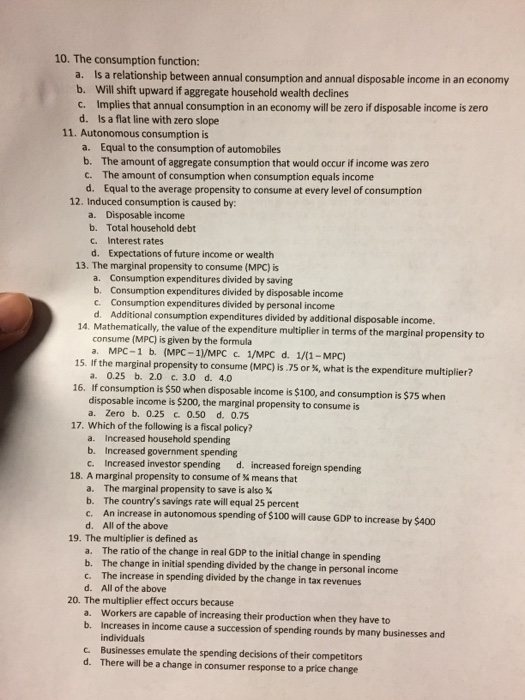### Create an Account

Home / Questions / The consumption function: Is a relationship between annual consumption and annual disposab...

# The consumption function: Is a relationship between annual consumption and annual disposable income is an economy Will shift upward if aggregate housed wealth declines Implies that annual consumption

The consumption function: Is a relationship between annual consumption and annual disposable income is an economy Will shift upward if aggregate housed wealth declines Implies that annual consumption in an economy will be zero if disposable income is zero Is a flat line with zero slope Autonomous consumption is Equal to the consumption of automobiles The amount of aggregate consumption that would occur if income was zero The amount of consumption when consumption equals income Equal to the average propensity to consume at every level of consumption Induced consumption is caused by: Disposable income Total household debt Interest rates Expectations of future income or wealth The marginal propensity to consume (MPC) is Consumption expenditures divided by saving Consumption expenditures divided by disposable income Consumption expenditures divided by personal income Additional consumption expenditures divided by additional disposable income Mathematically, the value of the expenditure multiplier in terms of the marginal propensity to consume (MPC) is given by the formula MPC - 1 (MPC - 1)/MPC 1/MPC 1/(1 - MPC) If the marginal propensity to consume (MPC) is .75 or %, what is the expenditure multiplier? 0.25 2.0 3.0 4.0 If consumption is \$50 when disposable income is \$100, and consumption is \$75 when disposable income is \$200, the marginal propensity to consume is Zero 0.25 0.50 0.75 Which of the following is a fiscal policy Increased household spending Increased government spending Increased investor spending Increased foreign spending A marginal propensity to consume of % means that The marginal propensity to save is also % The country's savings rate will equal 25 percent An increase in autonomous spending of \$100 will cause GDP to increase by \$400 All of the above The multiplier is defined as The ratio of the change in real GDP to the initial change in spending The change in initial spending divided by the change in personal income The increase in spending divided by the change in tax revenues All of the above The multiplier effect occurs because workers are capable of increasing their production when they have to Increase in income cause a succession of spending rounds by many business and individuals Business emulate the spending decisions of their competitors There will be a change in consumer response to a price changeJul 02 2021 View more View LessSubscribe To Get Solution